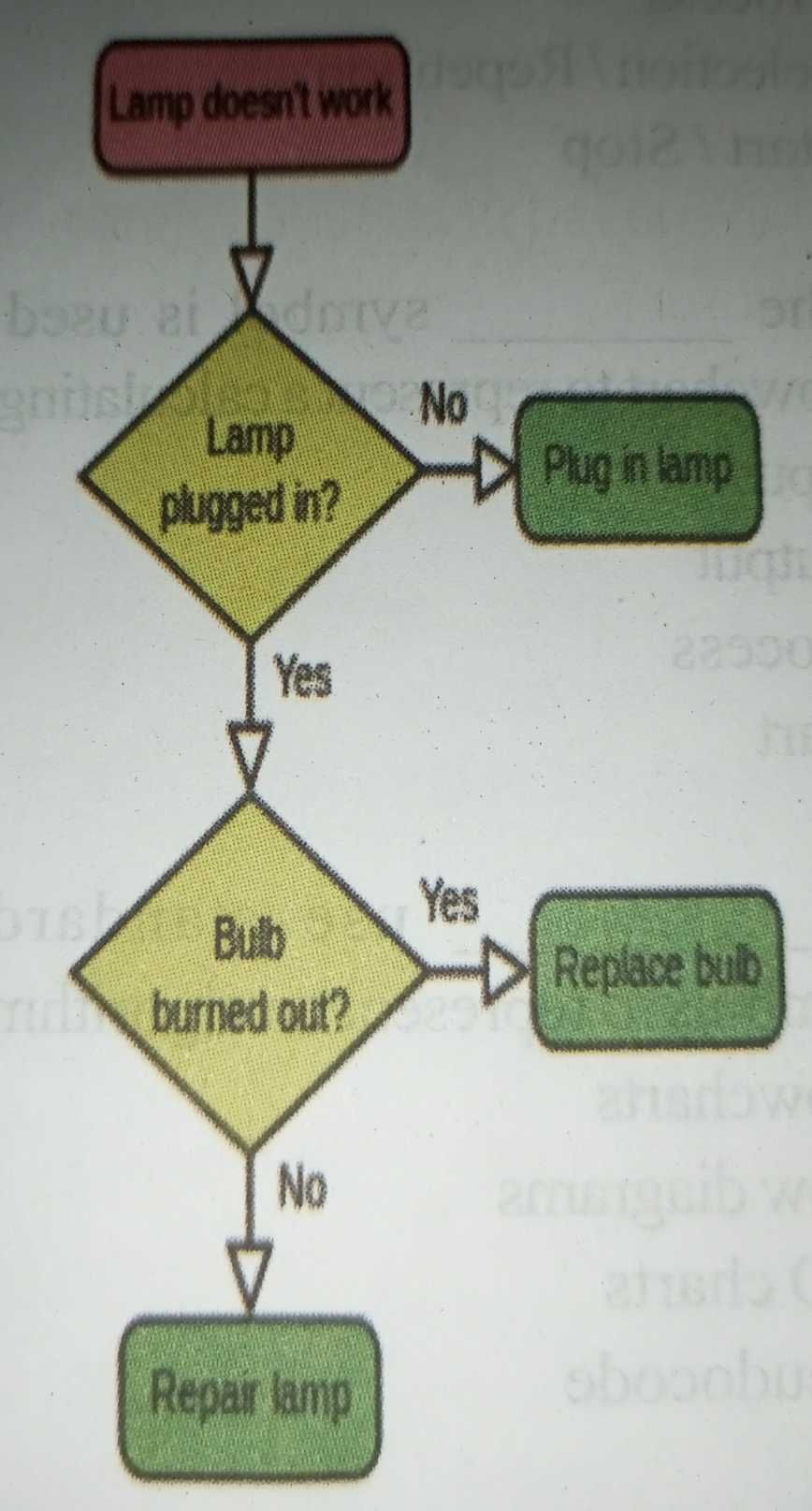Home » ALGORITHMS AND FLOWCHART

# ALGORITHMS AND FLOWCHARTALGORITHM

An algorithm is a set of instructions, sometimes called a procedure or a function that is used to perform a certain task.

Characteristics of an Algorithm

1. Finiteness: An algorithm terminates after a finite numbers of steps.
2. Definiteness: Each step in algorithm is unambiguous. This means that the action specified by the step cannot be interpreted (explain the meaning of) in multiple ways & can be performed without any confusion.
3. Input: An algorithm accepts zero or more inputs
4. Output: It produces at least one output.
5. Effectiveness: It consists of basic instructions that are realizable. This means that the instructions can be performed by using the given inputs in a finite amount of time.

FLOWCHART

A flowchart is a type of diagram that represents an algorithm or process, showing the steps as boxes of various kinds, and their order by connecting these with arrows. This diagrammatic representation can give a step-by-step solution to a given problem. Process operations are represented in these boxes, and arrows connecting them represent flow of control.

1. It provides an easy way of communication because any other person besides the programmer can understand the way they are represented.
2. It represents the data flow.
3. It provides a clear overview of the entire program and problem and solution.
4. It checks the accuracy in logic flow.
5. It documents the steps followed in an algorithm.
6. It provides the facility for coding.
7. It provides the way of modification of running program.
8. They show all major elements and their relationship.

LIMITATION OF FLOW CHARTS

1. It is the total perception of the designer who drawn the flowchart.
2. It is working differ from one compiler to another, one language to another in some time.
3. It needs well – defined requirements.
4. Its basically for structured programming.

BASIC FLOW CHART SYMBOLS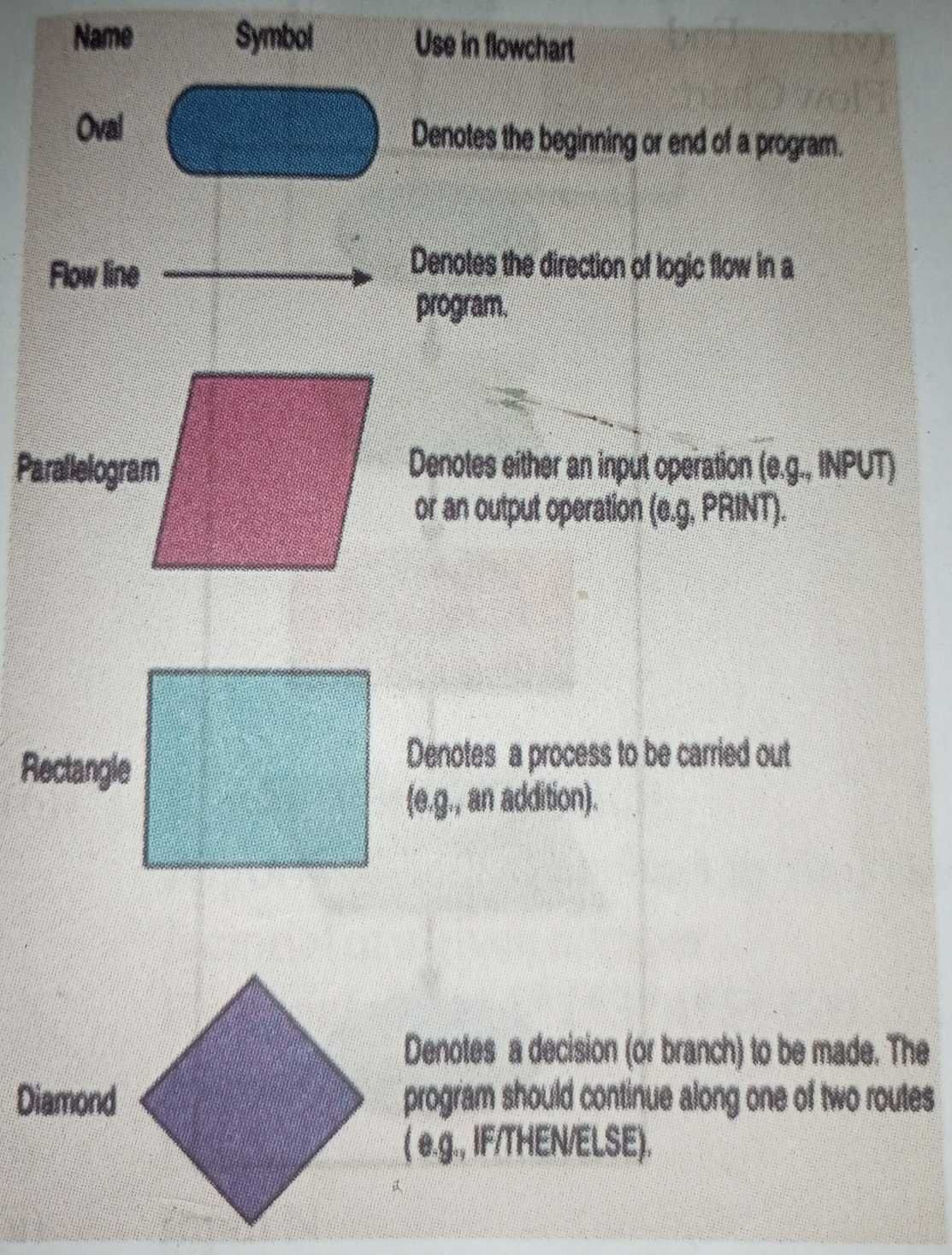Data flows are not typically represented in a flowchart, in contrast with data flow diagrams; rather, they are implied by the sequencing of operations. Flowcharts are used in analyzing, designing, documenting or managing a process or program in various fields.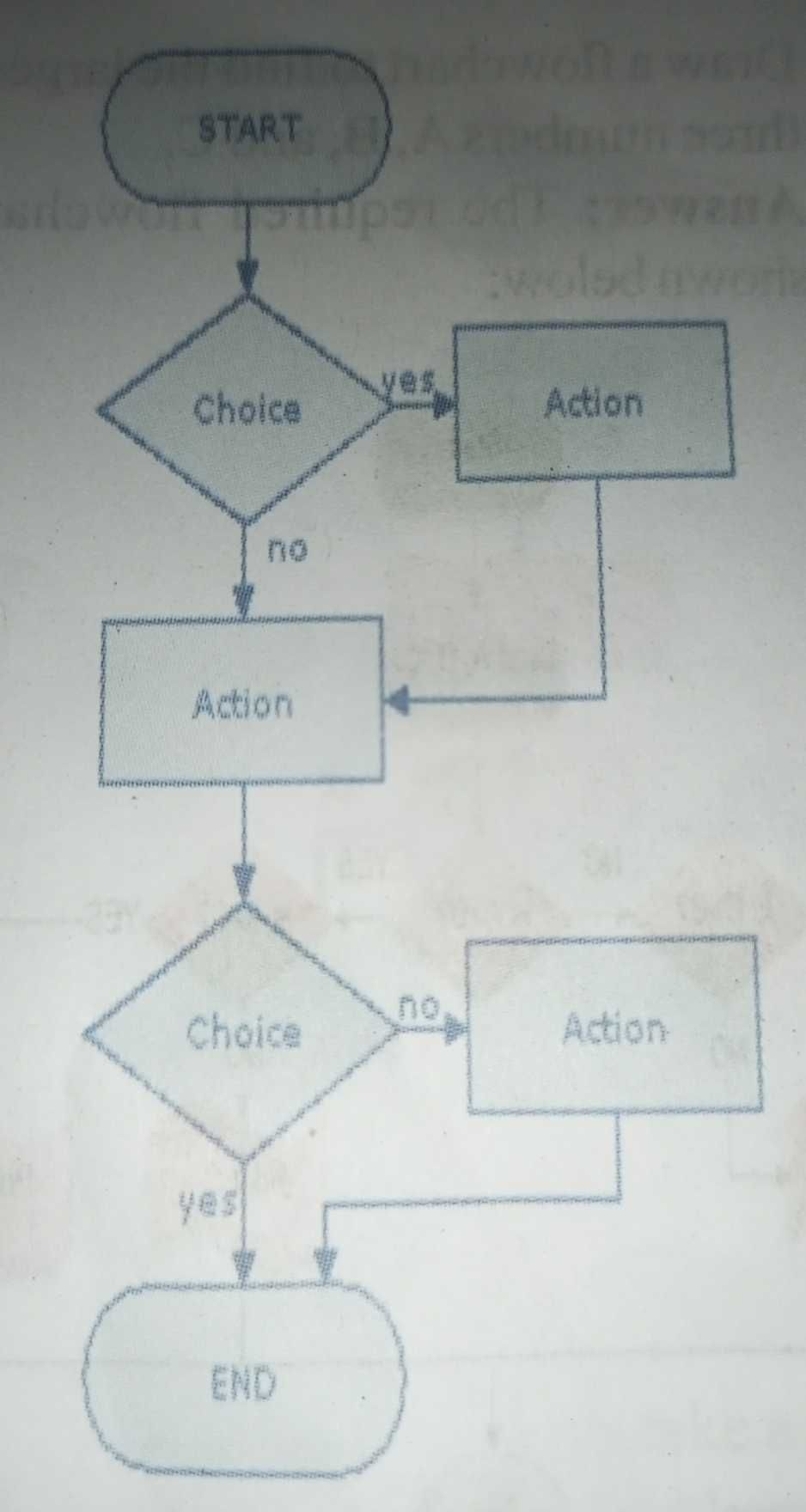FLOWCHART EXAMPLES

1) Draw a flowchart to find the sum of first 50 natural numbers.

Answer: The required flowchart is given below: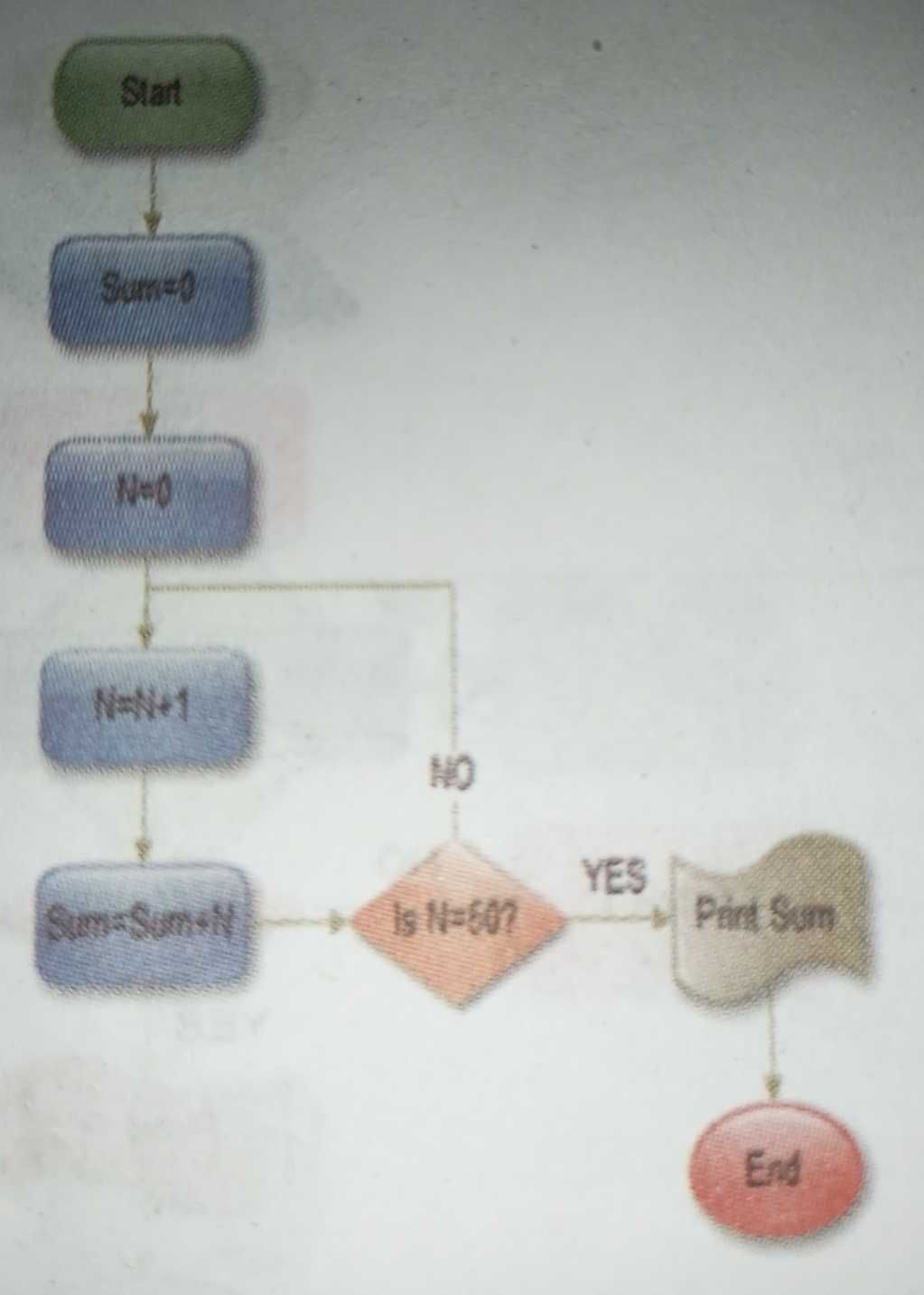2) Draw a flowchart to find the largest of three numbers A, B, and C.

Answer: The required flowchart is shown below: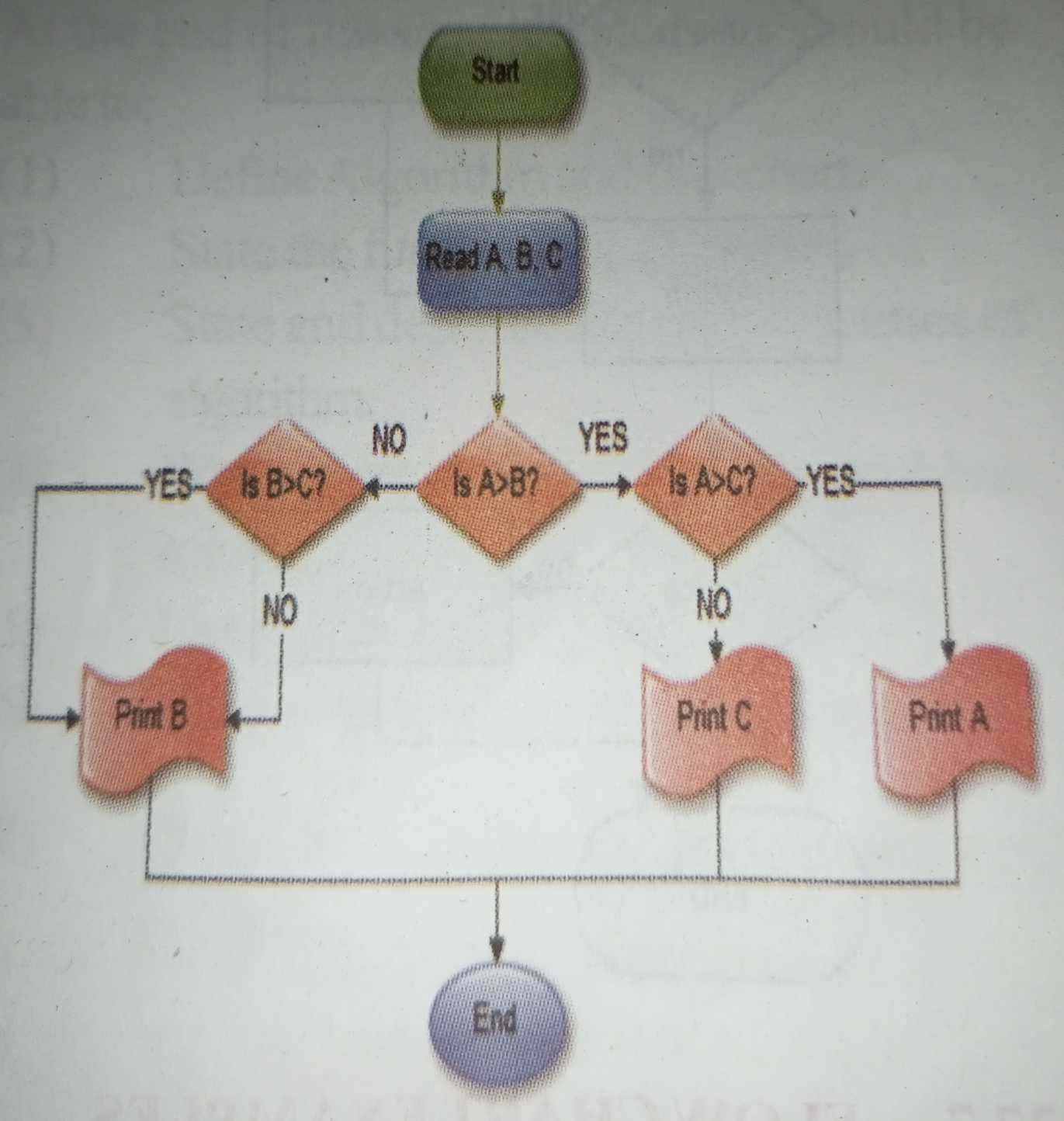3) Draw a flowchart for computing factorial

N (N!)

Where N! =1*2*3 *…. N

Answer: The required flowchart is shown below: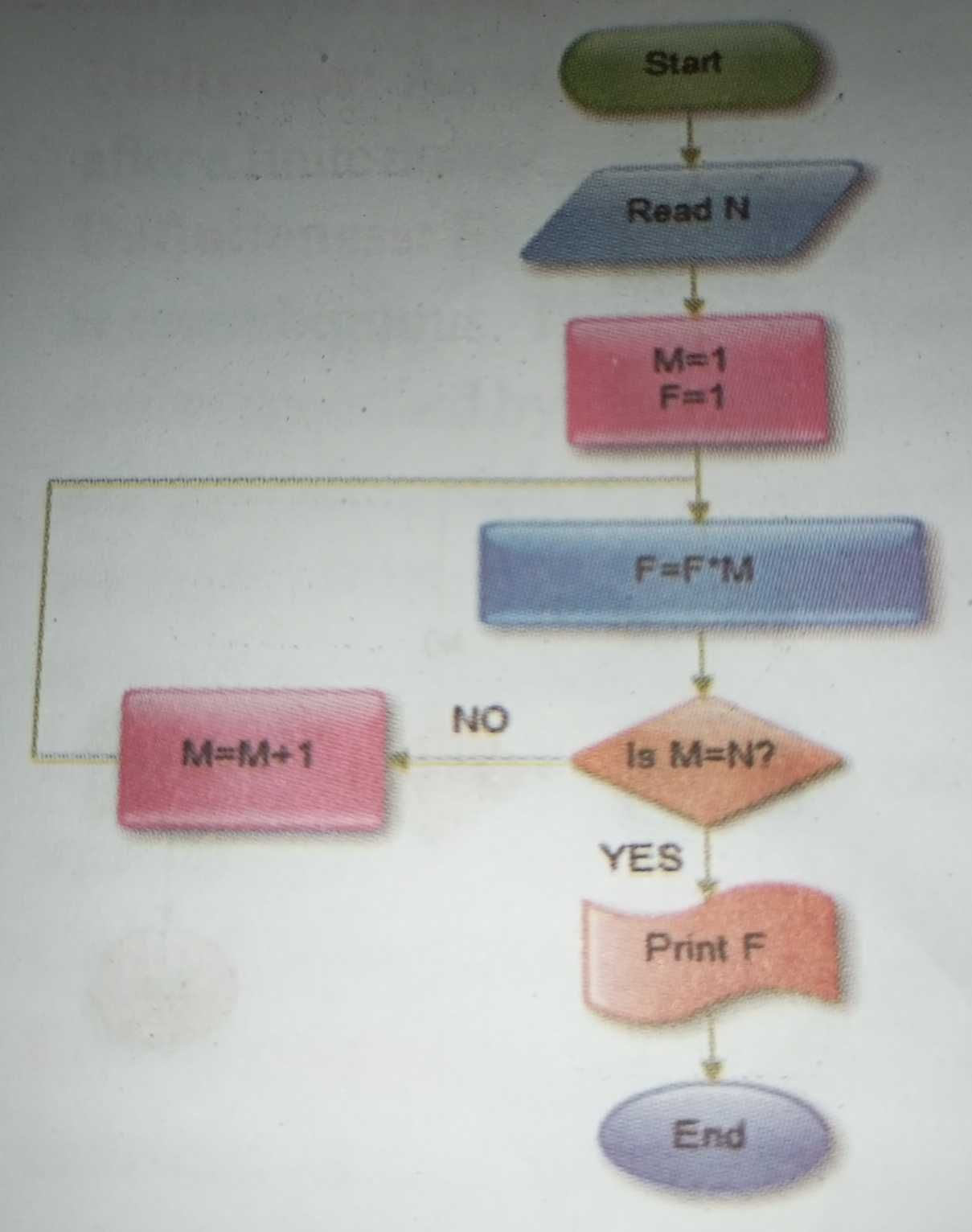4) Draw the Flow Chart and algorithm for Area and circumference of a Circle Formula:

(i) Area = πr²

(ii) Circumference = 2πr

(iii) r = radius of the circle.

Algorithm:

(i)  Begin

(ii) Input r

(iii) Area = π* r* r

(iv) Circumference= 2 * π * r

(v) Output Area, Circumference

(vi) End

Flow Chart: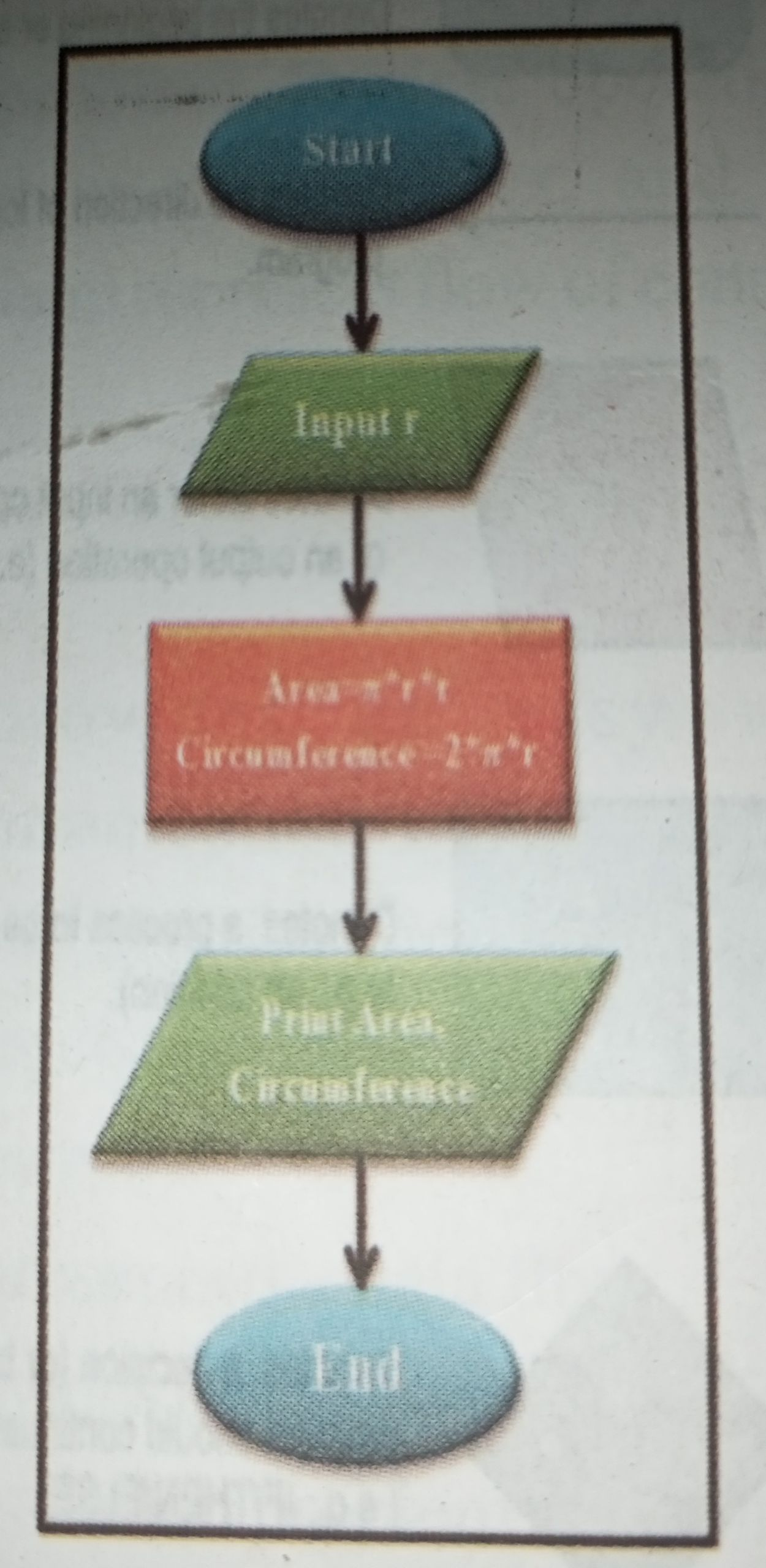(5) Draw the Flow Chart and algorithm for Product of first n natural numbers

Algorithm:

Begin

product =  1

for i        =  1 to n

Begin

product  =  product * i

End

Output product

End

Flow Chart: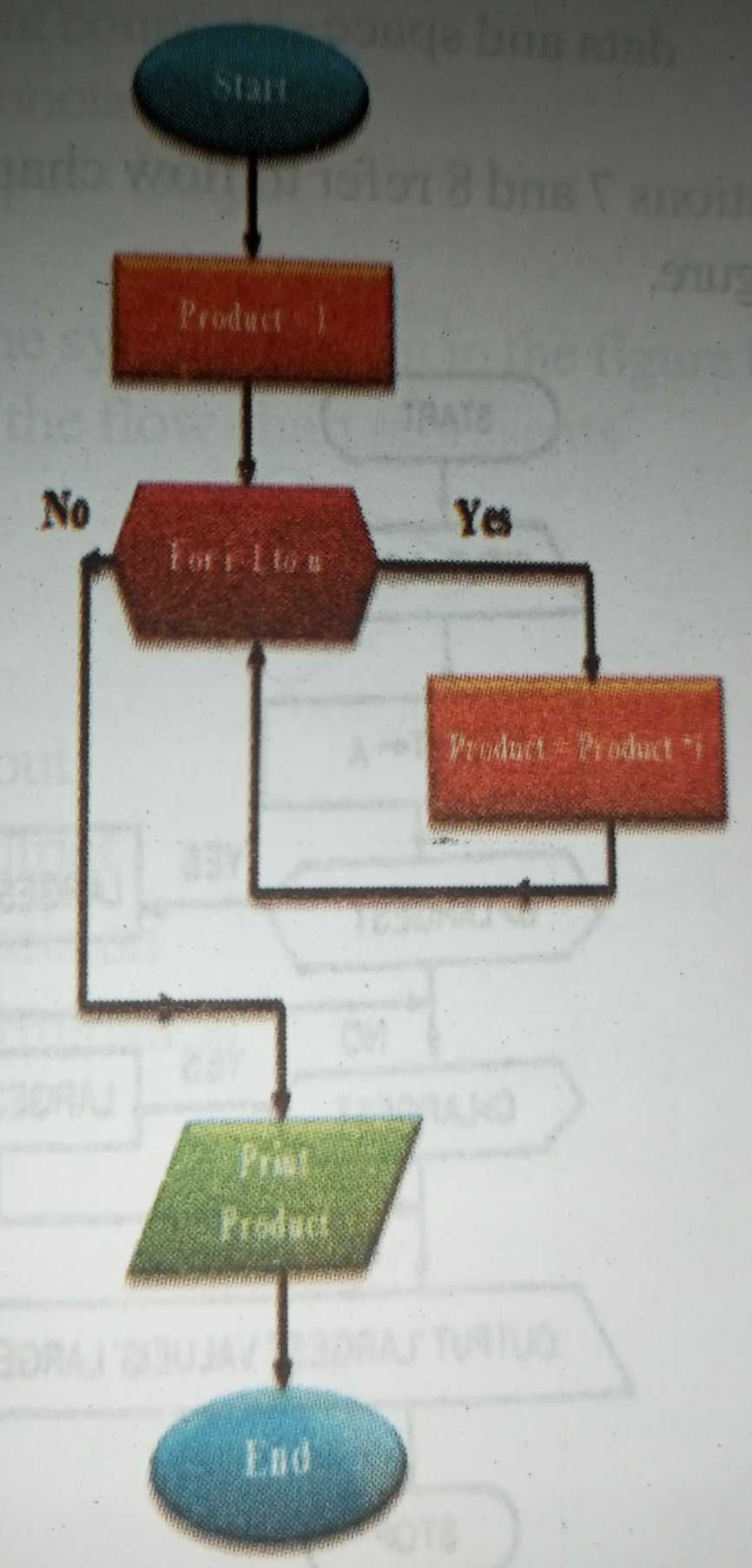6) Flow Chart for Factorial

Algorithm and flowchart to find the factorial of a given number

Factorial of 5 = 1*2*3*4*5=120

Algorithm:

(i) Begin

(i) Input N

(iii) Fact = 1

(iv) for i = 1 to N

(v) Begin

(vi) Fact = Fact *i

(vii) End

(viii) Output Fact

(ix) End

Flow chart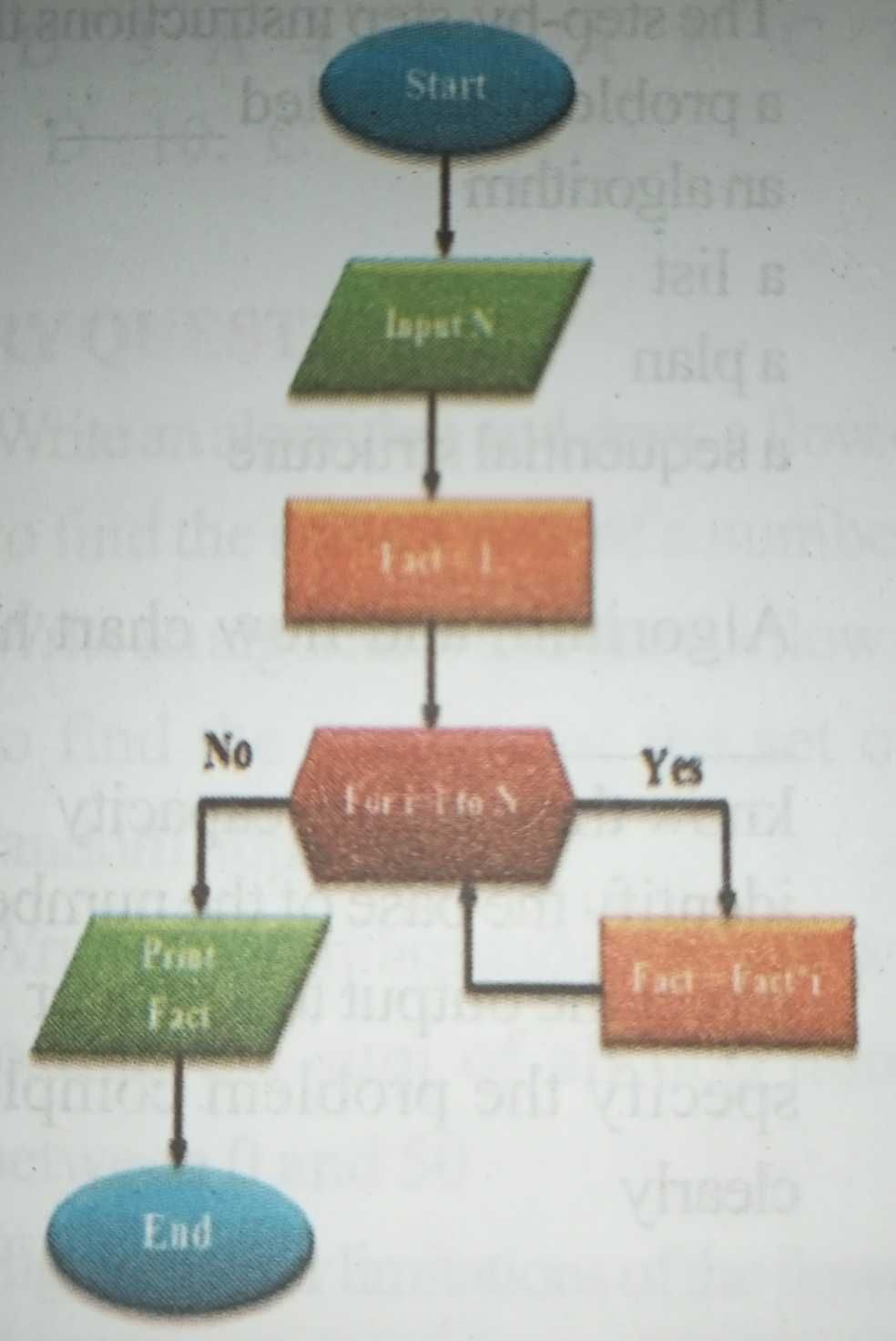7) Draw flow chart to take a decision on when to replace a bad lamp.